# Local outlier factor

What is the Local outlier factor?
Local outlier factor (LOF) is an algorithm used for Unsupervised outlier detection. It produces an anomaly score that represents data points which are outliers in the data set. It does this by measuring the local density deviation of a given data point with respect to the data points near it.

Working of LOF:
Local density is determined by estimating distances between data points that are neighbors (k-nearest neighbors). So for each data point, local density can be calculated. By comparing these we can check which data points have similar densities and which have a lesser density than its neighbors. The ones with the lesser densities are considered as the outliers.
Firstly, k-distances are distances between points that are calculated for each point to determine their k-nearest neighbors. The 2nd closest point is said to be the 2nd nearest neighbor to the point. Here is an image which represents k-distances of various neighbors in the cluster of a point: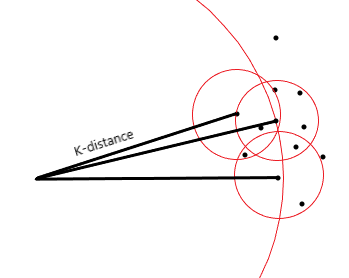This distance is used to calculate the reachability distance. It is defined as the maximum of the distance between two points and the k-distance of that point. Refer to the following equation, where B is the point in the center and A is a point near to it.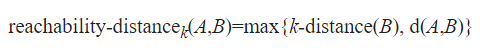Here is an image which represents reachability distance of a point to various neighbours: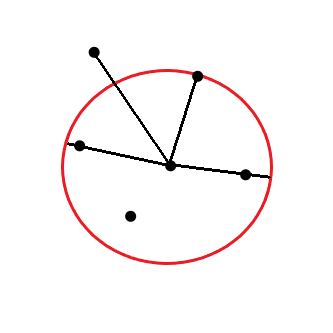As you can see in the image given above, for points inside the circle the k-distance is considered and for points outside the cluster, the distance between points is considered.
Now, reachability distances to all of the k-nearest neighbors of a point are calculated to determine the Local Reachability Density (LRD) of that point. The local reachability density is a measure of the density of k-nearest points around a point which is calculated by taking the inverse of the sum of all of the reachability distances of all the k-nearest neighboring points. The closer the points are, the distance is lesser, and the density is more, hence the inverse is taken in the equation.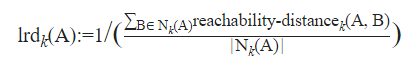The calculation of Local outlier factor (LOR) is done by taking the ratio of the average of the lrds of k number of neighbors of a point and the lrd of that point. Here is the equation for LOR: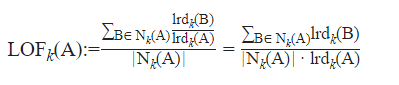So, in the equation, if the density of the neighbors and the point are almost equal we can say they are quite similar; if the density of the neighbors is lesser than the density of the point we can say the point is an inlier i.e. inside the cluster, and if the density of the neighbors is more than the density of the point we can say that the point is an outlier. Refer to the following illustration:

LOF ~ 1  =>  Similar data point

LOF < 1  =>  Inlier ( similar data point which is inside the density cluster)

LOF > 1  =>  Outlier

Here is an image of the plot of LOF on a data set: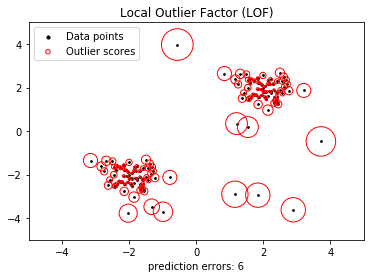• Sometimes it might get tricky to determine outliers. A point that is at a small distance from a very dense cluster might be considered as an outlier but a point that is at a farther distance from a wider spread cluster might be considered an inlier. With LOR, outliers in local areas are determined, so this issue does not persist.
• The method used in LOF can be applied in many other fields to solve problems of detecting outliers like geographic data, video streams, etc.
• The LOF can be used to implement a different dissimilarity function as well. And it is found to outperform many other algorithms of anamoly detection.

• It is not always the same LOF score that determines whether a point is an outlier or not. It might vary for different data sets.
• In higher dimensions, the LOF algorithm detection accuracy gets effected.
• As LOF score can be any number that the ratio produces, it might be a little inconvenient to understand the distinguishing of inliers and outliers based on it.
My Personal Notes arrow_drop_upCheck out this Author's contributed articles.

If you like GeeksforGeeks and would like to contribute, you can also write an article using contribute.geeksforgeeks.org or mail your article to contribute@geeksforgeeks.org. See your article appearing on the GeeksforGeeks main page and help other Geeks.

Please Improve this article if you find anything incorrect by clicking on the "Improve Article" button below.

Article Tags :
Practice Tags :

Be the First to upvote.

Please write to us at contribute@geeksforgeeks.org to report any issue with the above content.